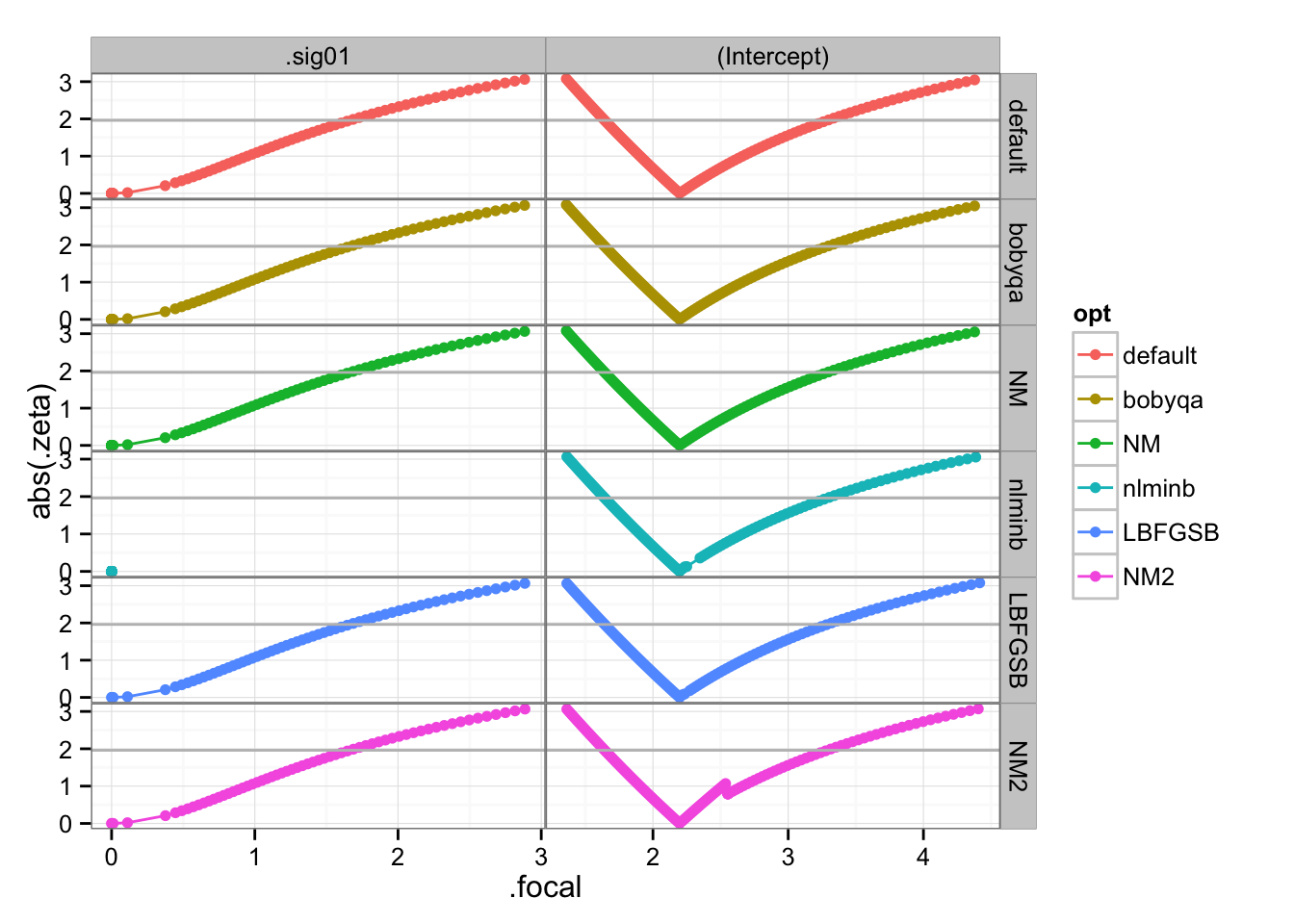Sophia Kyriakou asked whether she could ignore a whole bunch of warnings she was getting from lme4 when fitting models to (very small) simulations. tl;dr; yes. These are singular fits (due to a small number of levels of the grouping variable), but that’s not actually the proximal problem. It turns out that the second derivative of the random-effects std dev parameter is quite small near zero (the MLE), making the estimated uncertainty in this parameter very large and triggering warnings about scaling, etc etc, from lme4. Plotting the profiles demonstrates (for the most part) that the level of uncertainty is actually reasonable (upper 95% confidence interval around 2), just not well characterized by the local curvature at the MLE.

In the process I encountered (and hopefully alleviated) a number of issues of fragility with profiling. This is an unusual case in that the nloptr optimizers actually seem to perform worse than the built-in ones (with nloptr’s BOBYQA we can’t even successfully complete the profile). I haven’t figured out why nlminb failed – it worked on 32-bit Linux but fails here on 64-bit MacOS …) There’s also a little glitch (discontinuity) in the profile produced by nloptr’s Nelder-Mead implementation …

library("lme4")
library("optimx")
library("nloptr")
library("ggplot2")
library("grid") ## for unit()
theme_set(theme_bw()+theme(panel.margin=unit(0,"lines")))
library("lattice")

Note that I am using the very latest bleeding-edge lme4 version (i.e. Github version as of 13 Mar 2015 or later), which has slightly more robust profiling and allows for parallel computation of profiles.

Simulations: from Sophia Kyriakou (could also probably be done with simulate.merMod() …)

m <- 6
q <- 20
beta  <- 2
sigma2 <- 0.1
set.seed(206)
alpha <- rnorm(q, 0, sqrt(sigma2))
Y <- rbinom(q,m,plogis(alpha+beta))
cl <- seq.int(q)
tot <- rep(m,q)
dat <- data.frame(y = Y, tot = tot, cl = cl)
g0.default <- glmer(cbind(y, tot - y) ~ 1 + (1 | cl), data = dat,family =
binomial,nAGQ = 20)
## Warning in checkConv(attr(opt, "derivs"), opt$par, ctrl = control$checkConv, : Model is nearly unidentifiable: large eigenvalue ratio
##  - Rescale variables?
g0.bobyqa <- update(g0.default,
control=glmerControl(optimizer="bobyqa"))
## Warning in checkConv(attr(opt, "derivs"), opt$par, ctrl = control$checkConv, : Model is nearly unidentifiable: large eigenvalue ratio
##  - Rescale variables?
g0.NM <- update(g0.bobyqa,
control=glmerControl(optimizer="Nelder_Mead"))
## Warning in checkConv(attr(opt, "derivs"), opt$par, ctrl = control$checkConv, : Model is nearly unidentifiable: large eigenvalue ratio
##  - Rescale variables?
g0.nlminb <- update(g0.bobyqa,
control=glmerControl(optimizer="optimx",
optCtrl=list(method="nlminb")))
##
## Attaching package: 'minqa'
##
## The following objects are masked from 'package:nloptr':
##
##     bobyqa, newuoa
## Warning in optimx.check(par, optcfg$ufn, optcfg$ugr, optcfg$uhess, lower, : Parameters or bounds appear to have different scalings. ## This can cause poor performance in optimization. ## It is important for derivative free methods like BOBYQA, UOBYQA, NEWUOA. ## Warning in checkConv(attr(opt, "derivs"), opt$par, ctrl =
## control$checkConv, : Model failed to converge: degenerate Hessian with 1 ## negative eigenvalues g0.LBFGSB <- update(g0.bobyqa, control=glmerControl(optimizer="optimx", optCtrl=list(method="L-BFGS-B"))) ## Warning in checkConv(attr(opt, "derivs"), opt$par, ctrl =
## control$checkConv, : Hessian is numerically singular: parameters are not ## uniquely determined g0.bobyqa2 <- update(g0.bobyqa, control=glmerControl(optimizer="nloptwrap")) g0.NM2 <- update(g0.bobyqa, control=glmerControl(optimizer="nloptwrap", optCtrl=list(algorithm="NLOPT_LN_NELDERMEAD"))) ## Warning in checkConv(attr(opt, "derivs"), opt$par, ctrl =
## control\$checkConv, : Model failed to converge: degenerate Hessian with 1
## negative eigenvalues
fitList <- list(default=g0.default,
bobyqa=g0.bobyqa,
NM=g0.NM,
nlminb=g0.nlminb,
LBFGSB=g0.LBFGSB,
bobyqa2=g0.bobyqa2,  ## may fail profiling
NM2=g0.NM2)

Compare fits (differences in parameter estimates and negative log-likelihood relative to the default (bobyqa+N-M) optimizer combination):

ctab <- t(sapply(fitList,
function(x)
c(unlist(getME(x,c("theta","beta"))),
NLL=-logLik(x))))
(ctab2 <- sweep(ctab[-1,],ctab[1,],MARGIN=2,FUN="-"))
##         theta.cl.(Intercept)         beta          NLL
## bobyqa          1.578347e-04 5.592121e-09 8.881784e-15
## NM              2.423460e-05 5.890569e-09 0.000000e+00
## nlminb         -4.216526e-05 5.592123e-09 1.598721e-14
## LBFGSB         -4.216526e-05 2.452888e-08 1.421085e-14
## bobyqa2         7.221587e-03 1.151618e-05 3.879277e-09
## NM2            -4.216526e-05 5.592123e-09 1.598721e-14

Here we’re going to use lower delta (profile stepsize) parameter than default to get higher resolution of the profile.

profList <- lapply(fitList[names(fitList)!="bobyqa2"],
profile,dev.tol=1e-3,
delta=0.05,
parallel="multicore",
ncpus=3)
profdfList <-   Map(function(x,n) {
data.frame(as.data.frame(x),opt=n)
},
profList,names(profList))
profdf <- do.call(rbind,profdfList)
(gg1 <- ggplot(profdf,aes(.focal,abs(.zeta),colour=opt))+
geom_point()+geom_line()+facet_grid(opt~.par,scale="free")+
geom_hline(yintercept=1.96,col="gray"))